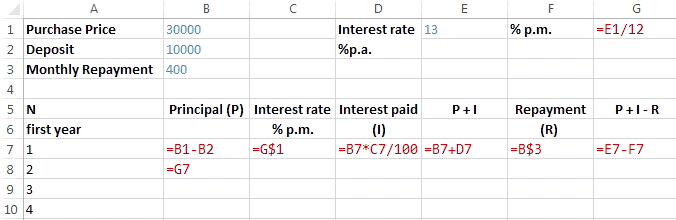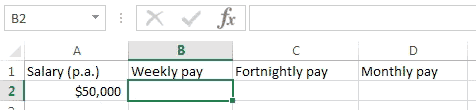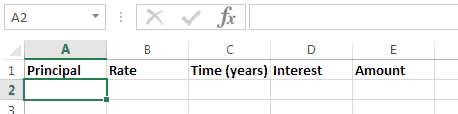### Excel formula

#### Reducible Interest | # gomaths.net/4264    User submitted, thanks to Amanda M

Calculate the reducible interest on a car loan.

B7: `=B1-B2`, C7: `=G\$1`, D7: `=B7*C7/100`, E7: `=B7+D7`

F7: `=B\$3`, G7: `=E7-F7`, B8: `=G7`#### Salary to pay | # gomaths.net/4218

Calculate weekly, fortnightly and monthly pay from a given salary.

`=A1/52` (for weekly)
`=A1/26` (for fortnightly)
`=A1/12` (for monthly)

where `A1` is the salary (per annum)#### Simple interest | # gomaths.net/4222

Calculate simple interest.

`=A2*B2*C2` (calculate simple interest)

`=A2+D2` (amount)

where `A2` is the Principal
`B2` is the interest rate as a percentage
`C2` is the time in years